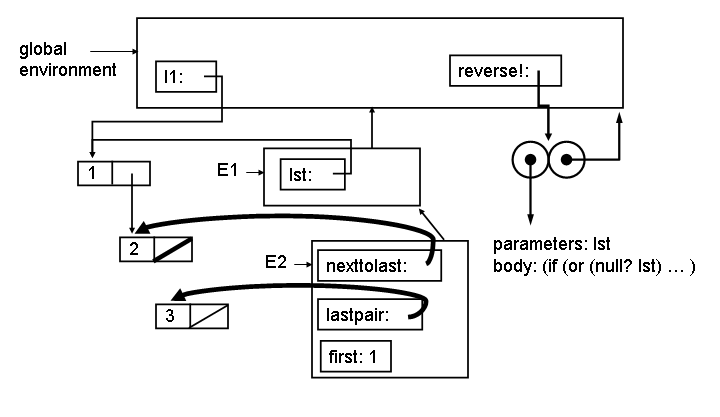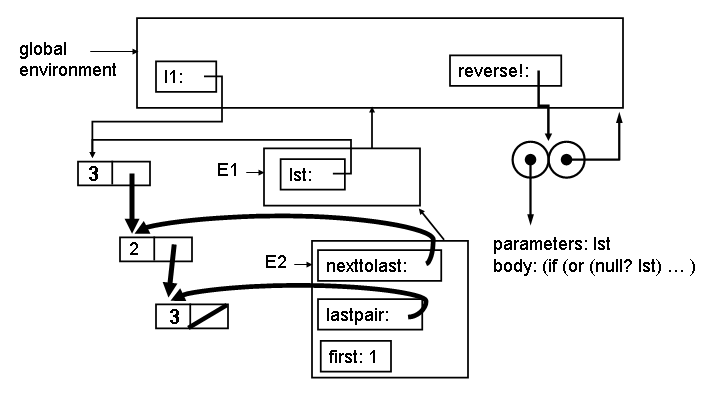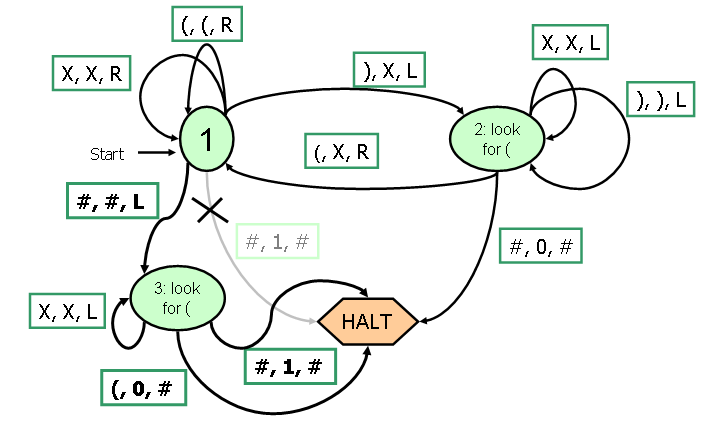University of Virginia, Department of Computer Science CS200: Computer Science, Spring 2002

 Final Exam - Answers and Discussion Out: 29 April 2002 Due: Monday, 6 May 2002, 5:00 PM (turn in your exam at Olsson 236A)

Each question is worth 10 points.

QuestionAverageQuestionAverage
110.0 29.8
36.3 48.1
57.3 69.3
77.0 87.2
98.7 108.6
Total82.3

### Procedures

1. Define a procedure increment-list that takes a list of numbers, and produces a new list of numbers with each number incremented by one. For example, (increment-list (list 2 0 0 88)) should evaluate to the list (3 1 1 89). Do not use map in your definition.

 ```(define (increment-list lst) (if (null? lst) lst (cons (+ 1 (car lst)) (increment-list (cdr lst))))) ```

2. Use map to define increment-list as in question 1.

 ```(define (increment-list lst) (map (lambda (x) (+ x 1)) lst)) ```

3. Define a procedure make-traced-procedure that can be used to observe applications of another procedure. Your make-traced-procedure should work like this:

> (define double (make-traced-procedure (lambda (x) (printf "in double~n") (+ x x))))

> (map double (list 1 2 3))

Called procedure
in double
(Result: 2)
Called procedure
in double
(Result: 4)
Called procedure
in double
(Result: 6)

(2 4 6)

Note that the traced procedure is only evaluated once (for each time it would be evaluated if it were not traced). You may use (display (list "Result: " val)) to print out the string "Result:" and the value val.

 Answer: Everyone found this question confusing — you needed to make sure the traced procedure is only evaluated once, and that the result of the call is the same as it would be without tracing. Here's how: ```(define (make-traced-procedure f) (lambda (x) (display "Called procedure") (let ((val (f x))) (display (list "Result: " val)) val))) ```

### Objects and Environments

Cy D. Fect defines a procedure reverse! that uses mutation to reverse a list:
```(define (next-to-last-pair lst)
(if (null? lst) (error "Null list!")
(if (null? (cdr lst)) (error "Single length list!")
(if (null? (cdr (cdr lst))) lst
(next-to-last-pair (cdr lst))))))

(define (reverse! lst)
(if (or (null? lst) (eq? 1 (length lst)))
#f ;; Nothing to do for empty or length 1 list (value doesn't matter)
(let* ((nexttolast (next-to-last-pair lst))
(lastpair (cdr nexttolast))
(first (car lst)))
;;; Point 0
(set-cdr! nexttolast null)
;;; Point 1
(reverse! (cdr lst))
(set-cdr! nexttolast lastpair)
(set-car! lst (car lastpair))
;;; Point 2
(set-car! lastpair first))))
```
Consider evaluating:

> (define ilist (list 1 2 3))

> (reverse! ilist)

> ilist

(3 2 1)

At Point 0 on the first call to reverse! the environment looks like:The E1 environment is created by the application (reverse! ilist). The E2 environment is created by the let inside reverse! (which desugars to an application of a lambda). (The let* actually desugars into more than one lambda, and more than one environment, but for this question we combine them into one environment.)

4. Show what the environment looks like at Point 1 (after evaluating (set-cdr! nexttolast null)). To make your drawing easier, here is a template drawing. You can answer this question by only adding to this template.

 The only thing that has changed is before Point 1 is (set-cdr! nexttolast null). This replaces the cdr part of the cons cell pointed to by nexttolast with null:5. Show what the environment looks like at Point 2. You can answer this question by only adding to this template.

 The call to reverse! reverses (cdr lst), which in this case is just the list (2) (since we previouly set the cdr to null). Then, we restore the pointer from the cdr the cons cell pointed to by nexttolast using (set-cdr! nexttolast lastpair) and replace the car of the first cons cell in the list with the car of the last. Note that the only thing left to do after point 2 is (set-car! lastpair first) which will correctly mutate the list into (3 2 1).### Turing Machines

6. The Turing Machine for checking balanced parentheses from Lecture 34 was incorrect. In particular, it would incorrectly report balanced parentheses for input such as ((). Correct the Turing Machine so it correctly ends with 1 at the tape head if the parentheses are balanced, and 0 otherwise. You should only need to add 1 state to the Turing Machine below:

 After we've crossed out all the right parens, we need to check the tape does not have any left parens. We do this by adding state 3, and replacing the old [#, 1 #] -> HALT transition from state 1 with [#, #, L] -> 3. In state 3, we keep moving left on X's, halt with 0 on the tape if we see a ( and halt with 1 on the tape if we reach the end of the tape.Russ noticed that the [), ), L] transition from state 2 was unnecessary!

### Lambda Calculus

7. Given T, F and if as defined in class:
T ≡ λ xy . x)
F ≡ λ xy . y)
if ≡ λ pca . pca
a. Define a Lambda Calculus term that behaves like not. For example, if (not T) M N should reduce to N and if (not F) M N should reduce to M.

 not ≡ λ qxy . qyx The easiest way to think about this is that T takes two parameters and reduces to the first parameter, F takes two parameters and reduces to the second parameter. We want something that swaps the meaning of T and F. Hence, we want to switch the order of the parameters. Another way to think about this is making not using if: not ≡ λ q . if q F T

b. Show that your definition of not works by showing the steps to reduce if (not T) M N to normal form (where M and N represent and Lambda Calculus term in normal form).

 if (not T) M N We know if does nothing (as long as it has three parameters), so can simplify right away to: (not T) M N Substituting our definition of not, we have ((λ qxy . qyx) T) M N Then, we β-reduce substituting T for q: ((λ xy . T yx)) M N Next, we β-reduce substituting M for x: ((λ y . T yM)) N Next, we β-reduce substituting N for y: T N M Note how we have reversed the order of the parameters to the T that was the parameter to not. Substituting our definition of T, we have (λ x (λ y . x)) N M Next, we β-reduce substituting N for x: (λ y . N)) M And finally, we β-reduce substituting M for y to get N: N

### Models of Computation

Phine Knight suggests the modelling computation using a Phine Machine consisting of a list of numbered instructions (the Instructions), a pointer to the current instructions (the InstructionPointer), and a store that associates name and values (the Store).

A program executes by executing the instruction numbered by the InstructionPointer, and then increasing the InstructionPointer by 1. This continues unless the Instruction is HALT.

Instructions can do one of four things:

• Set the value associated with a name to zero (e.g., X := 0)
• Set the value associated with a name to the value of a name plus 1 (e.g., Y := X - 1)
• Set the value associated with a name to the value of a name minus 1 (e.g., X := X - 1)
• Halt (e.g., HALT)
We can describe the state of a Phine Machine by listing the Instructions, InstructionPointer and Store. For example, here is a Phine Machine with four instructions and an empty store:
```   ( <1: X := 0
2: X := X + 1
3: Y := X - 1
4: HALT >,
1,
{ } )
```
The model will start by executing instruction number 1, which puts <X, 0> in the store and advances the Instruction Pointer to 2. After this, the state is:
```   ( <1: X := 0
2: X := X + 1
3: Y := X - 1
4: HALT >,
2,
{ <X, 0> } )
```
After the next two steps, the state will be:
```   ( <1: X := 0
2: X := X + 1
3: Y := X - 1
4: HALT>,
4,
{ <X, 1>, <Y, 0> } )
```

8. Write a BNF grammar that could be used to describe the state of a Phine Machine. A good answer will describe the smaller language possible that includes strings for describing all possible Phine Machine states.

 PhineMachine ::= ( Instructions, InstructionPointer, Store) Instructions ::= < InstructionList > InstructionList ::= Number : Instruction InstructionList InstructionList ::= Instruction ::= Name := 0 Instruction ::= Name := Name + 1 Instruction ::= Name := Name - 1 Instruction ::= HALT InstructionPointer ::= Number Store ::= { StoreItems } StoreItems ::= MoreStoreItems StoreItems ::= MoreStoreItems ::= StoreItem , MoreStoreItems MoreStoreItems ::= StoreItem StoreItem ::= < Name, Number>

9. Is this Phine Machine model of computation equivalent to a Turing Machine? Argue convincingly why it is or isn't.

 The Phine Machine is not equivalent to a Turing Machine, since it cannot model computations a Turing Machine can model. For example, there is no way to do an infinite loop with a Phine Machine, since it always just executes a finite list of instructions in order and has no way of modifying the instructions or jumping backwards in the instruction list. It is also clear that Phine Machine's have no way to make decisions.

10. Condy Shonal suggests adding one new instruction to Knight's model:

```    Instruction ::= IF Name = 0 GOTO Number
```
If the value of Name in the Store is 0, then this instruction sets the InstructionPointer to Number. If it is not, then this instruction does nothing and advances the InstructionPointer by one.

For example,

```    ( <1: IF A = 0 GOTO 5
2: B := B + 1
3: A := A - 1
4: GOTO 1
5: HALT>,
1,
{ <A, n>, <B, m> } )
```
is a program that will halt with B having the value n + m.

Condy claims her new model of computation is as powerful as a Turing Machine, but Fine Knight does not believe her. Write an informal but convincing argument that this model is as powerful as a Turing Machine.

 The Condy Machine is as powerful as a Turing Machine since it is powerful enough to simulate a Turing Machine. Informally, it can make decisions and jump using IF ... GOTO to model the state machine. It can keep track of the current state by using a value in the store, and change that state using the + 1 and - 1 instructions (as many times as necessary). It can model the tape using values in the store. It can use values in the store to model input and output. A more formal argument would need to show how to convert any TM into a Condy Machine. This would be really tricky, but it not necessary to make a convincing informal argument.

### Post Script

Your goal on this exam is to convince me you can think like a computer scientist. If you don't feel your answers to these questions will reflect fairly on your abilities, use this space to explain why. Otherwise, you don't need to write anything for this question.

 Here are some of the more interesting comments people wrote (which I won't credit, since I'm not sure everyone wants theirs to be known): Just a thought — if I were brave enough, I would've "unasked" all the exam questions and justified it with some grand discourse about logic and how thinking is so terrible anyway as the Zen masters claim. Instead, I did this exam the traditional way, using various languages that helped me to describe various computations. Attribute this option to my mastering the appropriateness of logic vs. language under a specific situation, or plain old common sense. Either way, I've gained some serious "how to" knowledge in this class. I never thought that I could ever understand computer science, but after this course I know how to do things that even my CS major friends and my father don't understand, and I've been able to apply what I've hearned to my [other] courses. I went from knowing a little HTML (blinking text!) to being able to decode the Lorenz Cipher - I think that's pretty good and I'm thirsty for more! Drinking from the firehose definitely game me brain damage at times, but I definitely got my fill! I think that making half the exam deal only with what we've learned in the last couple weeks of class is a little skewed. Out of nine students, I bet none get full credit on Problem 7. (True...but 3 got 9/10's. — Dave) Microsoft is the Devil Sneakers is the best movie ever. My dream is to be a cyborg, a perfect amalgamation of man and machine. Gödel's Incompleteness Theorem says that any axiomatic system is either incomplete or inconsistent. I think the same thing goes for a testing system that's aiming to test if someone can think like a computer scientist. Since testing such a thing is in the "interesting domain", its impossible to test all what we've learned in this class. I will play around with Scheme all night on Problem Sets (and I have definitely seen Thornton at 4am!) until I understand what it going on, but exams don't work the same way.